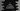# C program to check if a number is palindrome or not# C program to check if a number is palindrome or not :

A number is called a palindromic number if it remains same even if the digits are reversed. For example, 1123 is not a palindrome number but 12321 is a palindrome.

In this tutorial, we will learn how to check if a number is a palindrome or not in C. The user will enter the number and our program will check if it is a palindrome.

Basically, we will reverse the number and compare it with the original number. If both numbers are equal, that means it is a palindrome number. Else, it is not.

We have two different ways to check the palindrome of a number: using a while loop and by recursively. Let’s try to implement both methods one by one :

## Method 1: Using a while loop :

First of all, let’s implement the program using a while loop. The final program will look like below :

``````#include <stdio.h>
int main()
{
//1
int inputNumber;
int reverseNumber = 0;
int remainder;
int tempValue;

//2
printf("Enter a number: ");
scanf("%d", &inputNumber);

//3
tempValue = inputNumber;

//4
while (tempValue != 0)
{
//5
remainder = tempValue % 10;
reverseNumber = reverseNumber * 10 + remainder;
tempValue /= 10;
}

//6
if (reverseNumber == inputNumber)
{
printf("It is a palindrome number\n");
}
else
{
printf("It is not a palindrome number\n");
}
return 0;
}``````

### Explanation :

The commented numbers in the above program denote the step numbers below :

1. Create four integer variables to store different values in the program.
2. Ask the user to enter a number. Read and store it in the inputNumber variable.
3. Set the value of inputNumber to tempValue.
4. Run one while loop till the value of tempValue become 0.
5. Inside the loop reverse tempValue and store it in the reverseNumber.
6. Finally, check if the value of the reversed number and the original number is the same or not. If same, it is a palindrome number else not. Print out the result.

### Sample Output :

``````Enter a number: 1223
It is not a palindrome number

Enter a number: 1234321
It is a palindrome number``````

## Method 2: Using recursively :

This method is almost the same as the previous one. The only difference is that instead of using a while loop to find out the reverse of the number, we are using one different method to find out the reverse number recursively.

``````#include <stdio.h>
int reverse(int n)
{

static int reverseNum = 0, remainder;

if (n != 0)
{
remainder = n % 10;
reverseNum = reverseNum * 10 + remainder;
reverse(n / 10);
}

return reverseNum;
}

int main()
{
int inputNumber;
int reverseNumber;

printf("Enter a number: ");
scanf("%d", &inputNumber);

reverseNumber = reverse(inputNumber);

if (reverseNumber == inputNumber)
{
printf("It is a palindrome number\n");
}
else
{
printf("It is not a palindrome number\n");
}
return 0;
}``````

Here, reverse method takes one number and returns its reversed number. Then we are comparing that number to the original number.

### Sample Output :

``````Enter a number: 1222
It is not a palindrome number

Enter a number: 1456541
It is a palindrome number``````

## Conclusion :

We have learned two different ways to check if a number is a palindrome or not using C programming language in this tutorial. The output for both methods is the same. The only difference is that the second one calculates the reversed number recursively. Try to run these programs and drop one comment below if you have any queries.

You might also like :